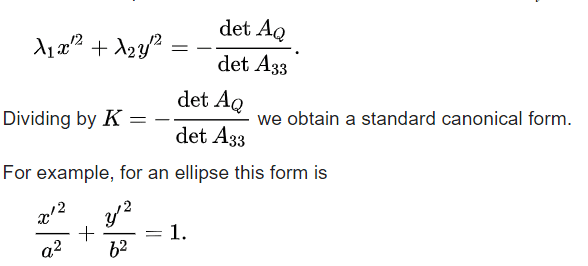# Canonical Form for quadratic equations *with* linear terms

• I
• arestes

#### arestes

TL;DR Summary
Not sure about the accepted "canonical form" for a quadratic equation WITH linear term
Hello:
I'm not sure if there's an accepted canonical form for a quadratic equation in two (or more) variables:
$$ax^2+by^2+cxy+dx+ey+f=0$$

Is it the following form? (using the orthogonal matrix Q that diagonalizes the quadratic part):

$$w^TDw+[d \ \ e]w+f=0$$
$$w^TDw+Lw+f=0$$
where
$$w=\begin{pmatrix} x' \\ y' \end{pmatrix} = Q^T \begin{pmatrix} x \\ y \end{pmatrix}$$
and
$$L=[d \ \ e]$$

Or is it a form with translated coordinates:
$$a(x'-m)^2+b(y'-n)^2+c(x'-m)(y'-n)+d(x'-m)+e(y'-n)+f'=0$$
with some to-be-determined constants m and n such that the linear terms vanish, which can be then used to change variables x'=x+m and y'=y+n.

I tried to find these m and n (expanding the binomials) but the simultaneous equations to satisfy in order to remove the linear terms are restricting and it seems to be impossible when $$c^2-4ab=0$$

Is it enough to leave the linear terms and call it "canonical form" just by diagonalizing the quadratic terms?

That's a neat condition (I haven't checked your work). ##c^2-4ab=0## means that ##D## is going to be singular, which people often want to exclude when talking about quadratic forms anyway.

In particular, suppose your quadratic form was ##x^2+y=0##. It's pretty clear you're not going to change coordinates to make this look nice, since it's just not quadratic in one direction.

My personal preference is the first one (simple scalar).

I think you're referring to Quadratic forms:
Yeah but quadratic forms don't have linear terms.

Thanks for reminding me of wikipedia. I did find the info I needed (almost) here:https://en.wikipedia.org/wiki/Matrix_representation_of_conic_sections

I found that there is a general form to write the equation of a conic (valid for central conics, which excludes parabolas because the K mentioned below doesn't exist):Here they call this equation "standard canonical form" but I'm not sure if it's THE canonical form.
My question still stands regarding the naming convention but I just realized that it's just that: semantics.

So, in the end (after learning stuff about projective geometry and homogeneous coordinates) is the name "canonical form" used for the "standard canonical" form above (although it doesn't work for parabolas for which, I guess it'll be just y^2=ax or x^2=ay)?